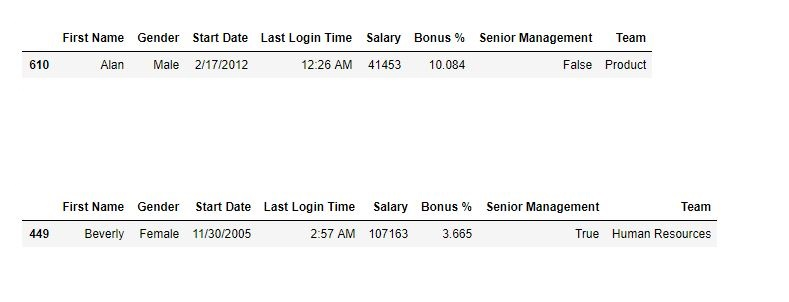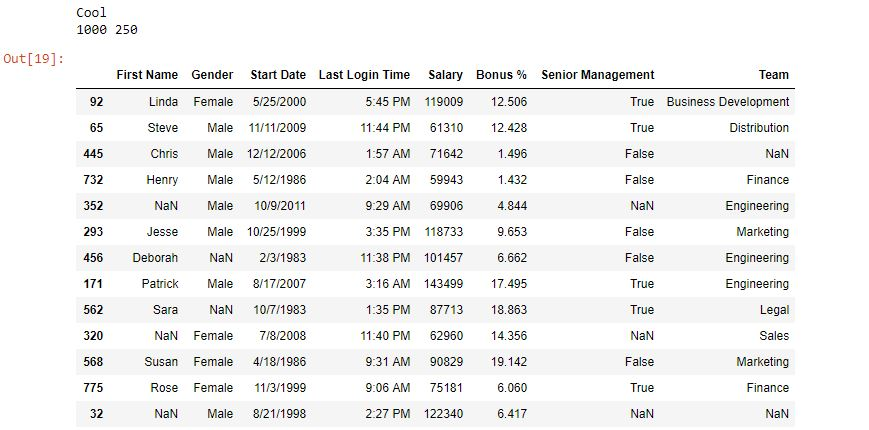# Python | Pandas Dataframe.sample()

• Difficulty Level : Easy
• Last Updated : 24 Apr, 2020

Python is a great language for doing data analysis, primarily because of the fantastic ecosystem of data-centric python packages. Pandas is one of those packages and makes importing and analyzing data much easier.

Pandas `sample()` is used to generate a sample random row or column from the function caller data frame.

Syntax:

DataFrame.sample(n=None, frac=None, replace=False, weights=None, random_state=None, axis=None)

Parameters:

n: int value, Number of random rows to generate.
frac: Float value, Returns (float value * length of data frame values ). frac cannot be used with n.
replace: Boolean value, return sample with replacement if True.
random_state: int value or numpy.random.RandomState, optional. if set to a particular integer, will return same rows as sample in every iteration.
axis: 0 or ‘row’ for Rows and 1 or ‘column’ for Columns.

Return type: New object of same type as caller.

Example #1: Random row from Data frame

In this example, two random rows are generated by the .sample() method and compared later.

 `# importing pandas package``import` `pandas as pd`` ` `# making data frame from csv file ``data ``=` `pd.read_csv(``"employees.csv"``)`` ` `# generating one row ``row1 ``=` `data.sample(n ``=` `1``)`` ` `# display``row1`` ` `# generating another row``row2 ``=` `data.sample(n ``=` `1``)`` ` `# display``row2`

Output:
As shown in the output image, the two random sample rows generated are different from each other.Example #2: Generating 25% sample of data frame
In this example, 25% random sample data is generated out of the Data frame.

 `# importing pandas package``import` `pandas as pd`` ` `# making data frame from csv file ``data ``=` `pd.read_csv(``"employees.csv"``)`` ` `# generating one row ``rows ``=` `data.sample(frac ``=``.``25``)`` ` `# checking if sample is 0.25 times data or not`` ` `if` `(``0.25``*``(``len``(data))``=``=` `len``(rows)):``    ``print``( ``"Cool"``)``    ``print``(``len``(data), ``len``(rows))`` ` `# display``rows`

Output:
As shown in the output image, the length of sample generated is 25% of data frame. Also the sample is generated randomly.My Personal Notes arrow_drop_up